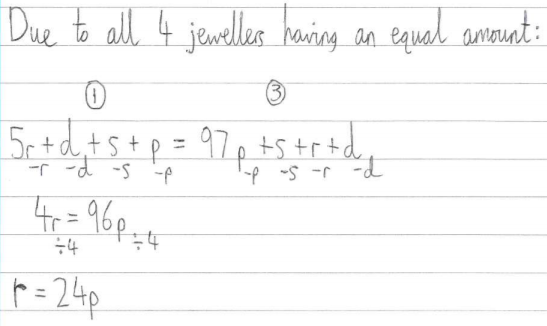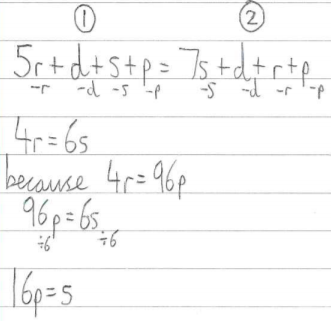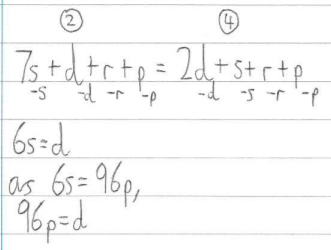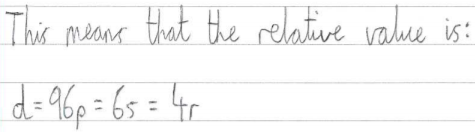#### You may also like### Golden Thoughts

Rectangle PQRS has X and Y on the edges. Triangles PQY, YRX and XSP have equal areas. Prove X and Y divide the sides of PQRS in the golden ratio.### At a Glance

The area of a regular pentagon looks about twice as a big as the pentangle star drawn within it. Is it?### Contact

A circular plate rolls in contact with the sides of a rectangular tray. How much of its circumference comes into contact with the sides of the tray when it rolls around one circuit?

##### Age 14 to 16Challenge Level

To work out how many of each type of gem each jeweller had, Fai from Bangkok Patana School used a diagram like this one, and this explanation (where $r, s , p, d$ represent the values of 1 ruby, 1 sapphire, 1 pearl and 1 diamond respectively):We have to subtract 3 gems from each jeweller because there are 4 jewellers and each jeweller gives one gem to each of the other jewellers.
Then we add one from each of the other 3 types of gem because we will gain one gem from each jeweller. For example the ruby jeweller had 8 rubies at the start then we subtract 3 rubies, which is 5 rubies then add one pearl, one diamond and one sapphire.
At the end we have the following expressions:
J1: $5r+p+d+s$
J2: $7s+r+p+d$
J3: $97p+d+s+r$
J4: $2d+s+r+p$

Simon from Sefton Park School in the UK displayed this nicely in a table:
 J1 J2 J3 J4 $5r$ $r$ $r$ $r$ $s$ $7s$ $s$ $s$ $p$ $p$ $97p$ $p$ $d$ $d$ $d$ $2d$

Frankie noticed that if you take 1 ruby, 1 sapphire, 1 pearl and 1 diamond from each jeweller, they will all still have collections of gems of equal value, so:
4 rubies = 6 sapphires = 96 pearls = 1 diamond

Amrit, Ray, James, Fai, Srushti, Archita and Alicia, Olivia & Bipanshu formed equations using pairs of expressions. This is Alicia, Olivia & Bipanshu's work, from the Ark Academy in the UK:Most people gave their answers in terms of $p$, like Amrit from Hymer's College in the UK, who wrote:
Using J1=J2, we have \begin{align}5r+s+p+d&=7s+r+p+d\\ 5r+s&=7s+r\\ 4r&=6s\\ r&=\frac{3}{2}s\end{align}
Using J2=J3, we have \begin{align}7s+r+p+d&=97p+r+s+d\\ 7s+p&=97p+s\\ 6s&=96p\\ s&=16p \hspace{5mm} \text{(or }p=\frac{1}{16}s\text{)}\end{align}
Using J3=J4, and substituting $p=\frac{1}{16}s$ and $r=\frac{3}{2}s$, we have
\begin{align}\frac{97}{16}s+\frac{3}{2}s+s+d&=\frac{3}{2}s+s+\frac{1}{16}s+2d\\ \frac{137}{16}s+d&=\frac{41}{16}s+2d\\ d&=6s\end{align}
Substituting $s=16p$ into $r=\frac{3}{2}s$ and $d=6s$, we get $d=96p$ and $r=24p.$ So the relative values of the gems in terms of $p$ are $p=p,$ $s=16p,$ $r=24p,$ $d=96p$

Raymond from Farm Cove Intermediate School in New Zealand, decided that:
Because $p$ is worth the least we will use J3 to compare to the others.
This gives $97p+r+s+d=5r+s+p+d$ simplifies to $96p=4r\implies r=24p$
$97p+r+s+d=7s+r+p+d$ simplifies to $96p=6s\implies s=16p$
$97p+r+s+d=2d+s+r+p$ simplifies to $96p=d$

Hamish from Watford Grammar School for Boys added pairs of expressions to form equations, and Lalit from Devonport High School for Boys subtracted pairs of expressions, and both solved the equations using a similar process.

Adithya from Hymers College and Liam from Grange Primary School in Australia used similar methods not involving algebra. This is Liam's explanation:

Each jeweller has 1 of each jewel with the addition of their original gems -3 {because they have given away 3 of their original gems}.

The ruby jeweller has 4 more rubies than anyone else {since the ruby jeweller has 5 rubies left and the others each have 1 ruby}, the sapphire jeweller has 6 extra, the pearl jeweller has 96 extra and the diamond jeweller has 1 extra diamond, meaning:
4 rubies = 6 sapphires = 96 pearls = 1 diamond
If you divide 96 by 4 you get 24. This means that 1 ruby is worth 24 pearls.
If you do this with every gem you will find 1 diamond is worth 96 pearls, 1 sapphire is worth 16 pearls and 1 ruby is worth 24 pearls.

Joel, Frankie and Zach all used algebraic versions of Liam's method. Frankie from the Ravensbourne School in the UK wrote:

$5r + 1s + 1p + 1d = 7s + 1r + 1p + 1d= 97p + 1r + 1s + 1d = 2d + 1r + 1s + 1p$
I decided to subtract $1s,$ $1p,$ $1r$ and $1d$ from all 4 {'sides' of the equation}:
$4r = 6s = 96p = 1d$

To work out how much each jeweller had lost or gained, Liam, James, Archita, Srushti, Ray and Alicia, Olivia & Bipanshu found the values of each collection in pearls to compare 'before' and 'after' values. Zach and Hamish both used a table to display the information clearly:

 Jeweller Start value in pearls End value in pearls Gain in pearls J1 $8r=192p$ $233p$ $233p-192p=41p$ J2 $10s=160p$ $233p$ $233p-160p=73p$ J3 $100p$ $233p$ $233p-100p=133p$ J4 $5d=480p$ $233p$ $233p-480p=-247p$

Fai, Joel and Frankie used their previous working to express the loss or gain of each jeweller in terms of the gems they had at the beginning. For example, Frankie had rearranged the equations and relative values to get

$s=\dfrac{2}{3}r,$ $p=\dfrac{1}{24}r$, $d=4r$
So J1 has: $5r+\dfrac{2}{3}r+\dfrac{1}{24}r+4r=\dfrac{233}{24}r$ worth of gems. {From $8r$, this is} a gain of $\dfrac{41}{24}r$, which is about $1.71r.$

Amrit and Lalit also found the changes correcly, Amrit by subtracting algebraic expressions, and Lalit assigning a value of 1000 to each collection. Well done to you all!